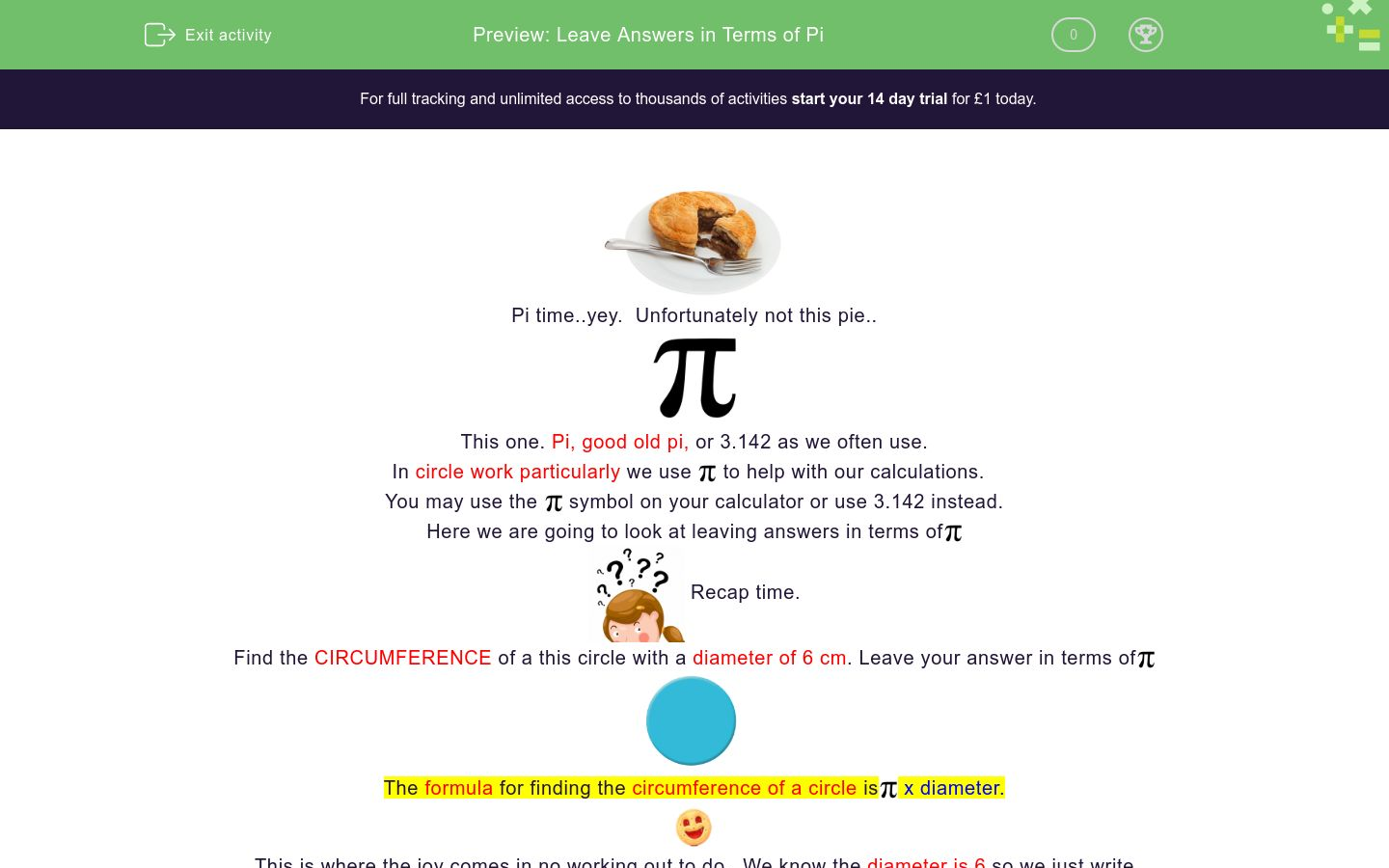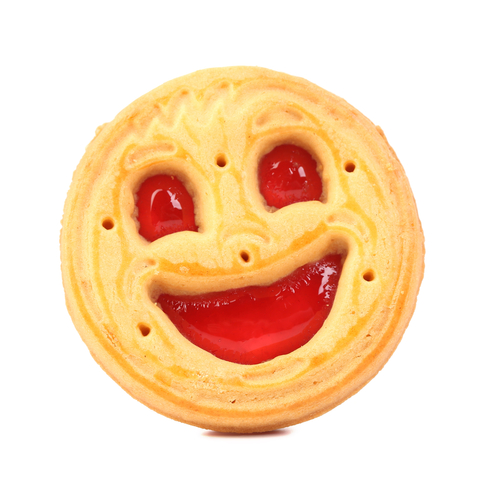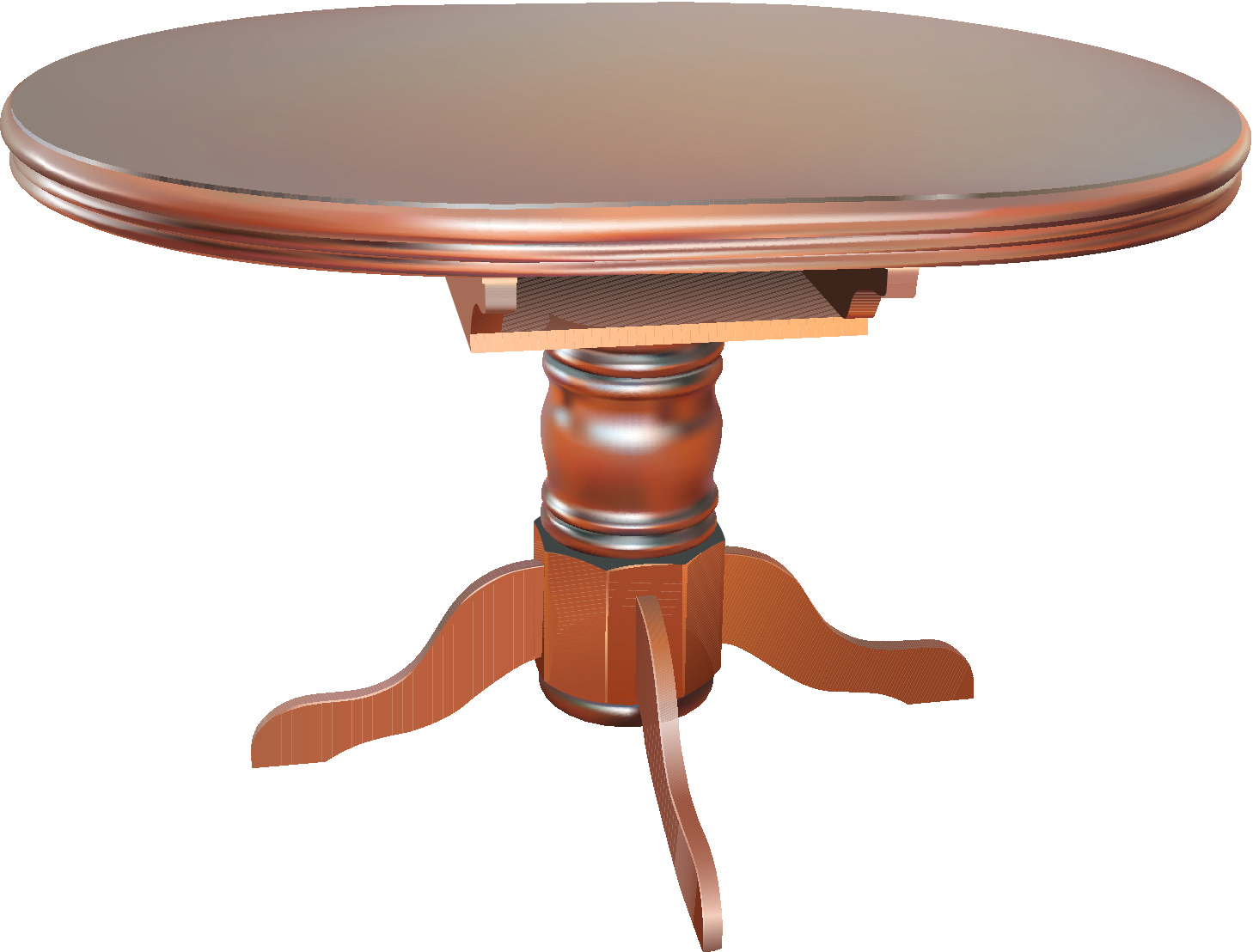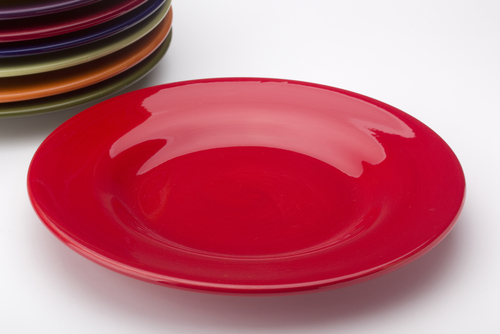# Leave Answers in Terms of Pi

In this worksheet, students will learn how to leave answers in terms of pi.Key stage:  KS 4

GCSE Subjects:   Maths

GCSE Boards:   AQA, Eduqas, Pearson Edexcel, OCR

Curriculum topic:   Geometry and Measures, Mensuration

Curriculum subtopic:   Mensuration and Calculation, Volume and Surface Area Calculations

Difficulty level:### QUESTION 1 of 10Pi time..yey.  Unfortunately not this pie..This one. Pi, good old pi, or 3.142 as we often use.

In circle work particularly we useto help with our calculations.

You may use thesymbol on your calculator or use 3.142 instead.

Here we are going to look at leaving answers in terms ofRecap time.

Find the CIRCUMFERENCE of a this circle with a diameter of 6 cm. Leave your answer in terms ofThe formula for finding the circumference of a circle isx diameter.This is where the joy comes in no working out to do.  We know the diameter is 6 so we just write

circumference = 6πFind the AREA of the circle with a radius of 3 cm

The formula for the area  of a circle =x radius x radius or  Πr²

Here all you have to do in find radius x radius and putafter it.

3 x 3 = 9 so my answer is 9π

You may be asked to calculate something a bit different and leave your answer in terms ofFor example, what is the length of the curve of this quarter circle.

You will have worked it all out and let's say you have an answer of 9.424777961.

To convert this into terms of Π just divide your answer by Π

It is best to use the Π button on your calculator as it will sometimes round it up for you.

Answers are usually shown to the nearest whole number.So our answer here is 3Π

Any calculation you have to show in terms of Π  just divide by ΠDid you know that 3.142% of sailors are pi rates!!!Find the circumference of this cake.

The diameter is 8 cm.

C = 24π cm

C = 16π cm

C = 8π cm

C = 4π cmFind the area of this coin.

π8 cm²

π4 cm²

π16 cm²

π12 cm²

Match up

## Column B

Circumference of a circle when diameter is 6 cm
36π cm²
Area of a circle with radius of 6 cm
12π cm
Circumference of a circle when diameter is 12 cm
6π cm
Circumference of a circle when diameter is 24 cm
49π cm²
Area of a circle with radius of 8 cm
24π cm
Area of a circle with diameter of 14 cm
64π cm²This dartboard has a diameter of 44 cm.

Calculate the following.  Leave you answers in terms of &pi:

## Column B

Circumference of a circle when diameter is 6 cm
36π cm²
Area of a circle with radius of 6 cm
12π cm
Circumference of a circle when diameter is 12 cm
6π cm
Circumference of a circle when diameter is 24 cm
49π cm²
Area of a circle with radius of 8 cm
24π cm
Area of a circle with diameter of 14 cm
64π cm²Calculate the circumference and area of this table in terms of Π

 60π cm 30π cm 120π cm 3600π cm² 360π cm² 36π cm² Circumference AreaThe area of this pond is 114 m²

What is its area in terms of Π

6Π cm²

36Π cm²

37&Pi cm²

6.6Π cm²The circumference of this pizza is  37.7 cm.

What is the circumference in terms of π

37Π cm

24Π cm

15Π cm

12Π cm

Match up to the answers in terms of Π

Round to the nearest whole number,

## Column B

Circumference 98 cm
48Π cm²
Circumference 44 cm
14Π cm
Circumference 56 cm
17Π cm
Area 42 cm²
13Π cm²
Area 150 cm²
31Π cm
Area 88 cm²
28Π cm²The blue circle has a diameter of 12 cm and a the green circle has a diameter of 4 cm.

Answers have been rounded to 2 decimal places.

 37. 60cm 37.70 cm 12.57cm 12.66 cm 52.36 cm 50. 27 cm 25π 16π Circumference of the blue circle Circumference of the the green circle Total Circumference for both circles Total circumference for both circles in terms of πThis crockery set has a plate with a radius of 15 cm and a saucer with a radius of 7 cm.

Answers have been rounded to 2 decimal places. Calculate the

 620.12cm² 706.95 cm² 153.96 cm² 155.65 cm² 860.91 cm² 680.91 cm² 276π 274Π Area of the plate Area of the saucer Total Area of plate and saucer Total area in terms of π
• Question 1Find the circumference of this cake.

The diameter is 8 cm.

C = 8π cm
EDDIE SAYS
Oh how nice, no working out to do. Take π and put the number for the diameter in front of it. It's just like having your cake and eating it. Circumference = 8π
• Question 2Find the area of this coin.

π4 cm²
EDDIE SAYS
The formula for finding area = π x r x r r x r = 4 so area = 4π How easy is that, no worries about 3.142 and very little working out. Bargain.
• Question 3

Match up

## Column B

Circumference of a circle when di...
6π cm
Area of a circle with radius of 6...
36π cm²
Circumference of a circle when di...
12π cm
Circumference of a circle when di...
24π cm
Area of a circle with radius of 8...
64π cm²
Area of a circle with diameter of...
49π cm²
EDDIE SAYS
Hopefully you are now getting into the swing of things. Circumference is always diameter x Π e.g circumference = 6π Area just calculate the radius x radius first and put π after it. e.g 6 x 6 = 36 area = 36π Did you spot the sneaky one? Of course you did one area gave the diameter and you had to halve it to get the radius. No much gets past you.
• Question 4This dartboard has a diameter of 44 cm.

Calculate the following.  Leave you answers in terms of &pi:

EDDIE SAYS
Once you know the diameter the rest is easy. Circumference = 44Π Area find the radius first 44 ÷ 2 = 22 22 x 22 = 484 Area = 484Π
• Question 5Calculate the circumference and area of this table in terms of Π

 60π cm 30π cm 120π cm 3600π cm² 360π cm² 36π cm² Circumference Area
EDDIE SAYS
Did you spot it? Of course, you were given the radius and not the diameter. For the circumference you needed to double 60cm (120cm) For the Area 60 x 60 = 3600 It is always worth just stopping to write down formulas and see what information you are already given. Any information you are not given can be worked out like in this question. To get a diameter we double the radius.
• Question 6The area of this pond is 114 m²

What is its area in terms of Π

36Π cm²
EDDIE SAYS
Now this is where the Π button on your calculator comes into its own. Answers in terms of Π are nearly always rounded to the nearest whole number. Some calculators do this automatically. If you are going to use 3.142 round up to the nearest whole number unless otherwise told. Divide your area by Π that is all there is to it. 114 ÷ Π = 36Π cm²
• Question 7The circumference of this pizza is  37.7 cm.

What is the circumference in terms of π

12Π cm
EDDIE SAYS
You can't eat it until you have calculated the circumference. Simple just divide by &Pi. 37.7 ÷ π = 12π cm
• Question 8

Match up to the answers in terms of Π

Round to the nearest whole number,

## Column B

Circumference 98 cm
31Π cm
Circumference 44 cm
14Π cm
Circumference 56 cm
17Π cm
Area 42 cm²
13Π cm²
Area 150 cm²
48Π cm²
Area 88 cm²
28Π cm²
EDDIE SAYS
If you have had to calculate something and convert the answer in terms of π this is the easy bit. All the hard work you have done before. Take your answer and divide by π Answers are usually given to the nearest whole number.
• Question 9The blue circle has a diameter of 12 cm and a the green circle has a diameter of 4 cm.

Answers have been rounded to 2 decimal places.

 37. 60cm 37.70 cm 12.57cm 12.66 cm 52.36 cm 50. 27 cm 25π 16π Circumference of the blue circle Circumference of the the green circle Total Circumference for both circles Total circumference for both circles in terms of π
EDDIE SAYS
Were you going around in circles? This is just a combination of your skill set so far. Circumference = π x diameter. Use this to find circumferences of both circles. Add together to get total circumference Divide by π to get total circumference in terms of π
• Question 10This crockery set has a plate with a radius of 15 cm and a saucer with a radius of 7 cm.

Answers have been rounded to 2 decimal places. Calculate the

 620.12cm² 706.95 cm² 153.96 cm² 155.65 cm² 860.91 cm² 680.91 cm² 276π 274Π Area of the plate Area of the saucer Total Area of plate and saucer Total area in terms of π
EDDIE SAYS
Are you feeling pi eyed from all this? This is just a combination of your skill set so far. Area = π x r x r. Use this to find area of plate and saucer Add together to get total area Divide by π to get total area in terms of π I need a strong cup of tea after that.
---- OR ----

Sign up for a £1 trial so you can track and measure your child's progress on this activity.

### What is EdPlace?

We're your National Curriculum aligned online education content provider helping each child succeed in English, maths and science from year 1 to GCSE. With an EdPlace account you’ll be able to track and measure progress, helping each child achieve their best. We build confidence and attainment by personalising each child’s learning at a level that suits them.

Get started